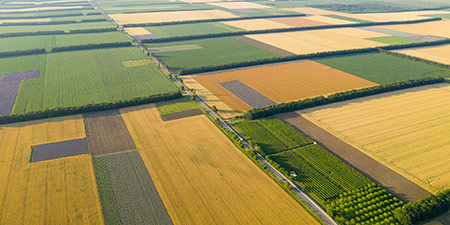# Square Foot (ft²) to Square Meter (m²)

Area Units ﹣ Converter Square Foot to Square Meter

Here you can convert the Area unit Square Foot into the unit Square Meter and vice versa you can convert Square Meter into Square Foot. By clicking the "Swap units" icon, you will always obtain the desired conversion in the calculation result, i.e., ft² to m² or m² to ft². With the following calculator you can also calculate any other Area unit.

A square foot (ft²; plural square feet) is equal to the area of a square with a side length of one foot (30.48 cm).

1 ft² = 1 ft × 1 ft

1 ft² = 30,48 cm × 30,48 cm

A foot is 12 inches long in the Anglo-American system and thus a square foot has 144 square inches. In the Anglo-American world, the size of land is indicated in land and property surveys using only the two area units, acre and square foot.

One square meter (m²; spelled metre in Commonwealth English and meter in American English) is equal to the area of a square with a side length of one meter.

1 m² = 1 m × 1 m

The square meter is the base unit for area in the International System of Units (SI) and in other metric systems. All SI-compliant units of area are derived from the meter and its parts and multiples. Often, instead of the abbreviation "m²", the (obsolete) abbreviation "qm" is still used for the square meter.

Typical orders of magnitude, which are measured or represented in square meters, are for example the skin surface of a human being with approximately 1.5 to 2 m², the size of a tennis court with 260 m² or property sizes with e.g. 400 m².

## Basis for conversion Square Foot (ft²) to Square Meter (m²) and vice versa

The abbreviation for the "Area unit Square Foot" is ft². The abbreviation for the "Area unit Square Meter" is m².

## Formula for the conversion of Square Foot (ft²) to Square Meter (m²) and vice versa

The calculation from Square Foot to Square Meter shall be made using the following conversion formula:

Conversion formula Square Foot to Square Meter

Determine the number of Square Meter from Square Foot

Square Foot × 0.09290304

## Formula for the conversion of Square Meter (m²) to Square Foot (ft²)

The calculation from Square Meter to Square Foot shall be made using the following conversion formula:

Conversion formula Square Meter to Square Foot

Determine the number of Square Foot from Square Meter

Square Meter × 10.76391041671

## Overview table : How many Square Foot are in a Square Meter ?

Square Foot ft² ⇒ Square Meter m²
0.01 ft²  are  0.00092 m²
0.02 ft²  are  0.00185 m²
0.03 ft²  are  0.00278 m²
0.04 ft²  are  0.00371 m²
0.05 ft²  are  0.00464 m²
0.06 ft²  are  0.00557 m²
0.07 ft²  are  0.00650 m²
0.08 ft²  are  0.00743 m²
0.09 ft²  are  0.00836 m²
0.10 ft²  are  0.00929 m²
0.20 ft²  are  0.01858 m²
0.30 ft²  are  0.02787 m²
0.40 ft²  are  0.03716 m²
0.50 ft²  are  0.04645 m²
0.60 ft²  are  0.05574 m²
0.70 ft²  are  0.06503 m²
0.80 ft²  are  0.07432 m²
0.90 ft²  are  0.08361 m²
1 ft²  corresponds to  0.09290 m²
2 ft²  are  0.18580 m²
3 ft²  are  0.27870 m²
4 ft²  are  0.37161 m²
5 ft²  are  0.46451 m²
6 ft²  are  0.55741 m²
7 ft²  are  0.65032 m²
8 ft²  are  0.74322 m²
9 ft²  are  0.83612 m²
10 ft²  are  0.92903 m²
20 ft²  are  1.85806 m²
30 ft²  are  2.78709 m²
40 ft²  are  3.71612 m²
50 ft²  are  4.64515 m²
60 ft²  are  5.57418 m²
70 ft²  are  6.50321 m²
80 ft²  are  7.43224 m²
90 ft²  are  8.36127 m²
100 ft²  are  9.29030 m²
200 ft²  are  18.58060 m²
300 ft²  are  27.87091 m²
400 ft²  are  37.16121 m²
500 ft²  are  46.45152 m²
600 ft²  are  55.74182 m²
700 ft²  are  65.03212 m²
800 ft²  are  74.32243 m²
900 ft²  are  83.61273 m²
1 000 ft²  are  92.90304 m²

## Overview table : How many Square Meter are in a Square Foot ?

Square Meter m² ⇒ Square Foot ft²
0.01 m²  are  0.10763 ft²
0.02 m²  are  0.21527 ft²
0.03 m²  are  0.32291 ft²
0.04 m²  are  0.43055 ft²
0.05 m²  are  0.53819 ft²
0.06 m²  are  0.64583 ft²
0.07 m²  are  0.75347 ft²
0.08 m²  are  0.86111 ft²
0.09 m²  are  0.96875 ft²
0.10 m²  are  1.07639 ft²
0.20 m²  are  2.15278 ft²
0.30 m²  are  3.22917 ft²
0.40 m²  are  4.30556 ft²
0.50 m²  are  5.38195 ft²
0.60 m²  are  6.45834 ft²
0.70 m²  are  7.53473 ft²
0.80 m²  are  8.61112 ft²
0.90 m²  are  9.68751 ft²
1 m²  corresponds to  10.76391 ft²
2 m²  are  21.52782 ft²
3 m²  are  32.29173 ft²
4 m²  are  43.05564 ft²
5 m²  are  53.81955 ft²
6 m²  are  64.58346 ft²
7 m²  are  75.34737 ft²
8 m²  are  86.11128 ft²
9 m²  are  96.87519 ft²
10 m²  are  107.63910 ft²
20 m²  are  215.27820 ft²
30 m²  are  322.91731 ft²
40 m²  are  430.55641 ft²
50 m²  are  538.19552 ft²
60 m²  are  645.83462 ft²
70 m²  are  753.47372 ft²
80 m²  are  861.11283 ft²
90 m²  are  968.75193 ft²
100 m²  are  1 076.39104 ft²
200 m²  are  2 152.78208 ft²
300 m²  are  3 229.17312 ft²
400 m²  are  4 305.56416 ft²
500 m²  are  5 381.95520 ft²
600 m²  are  6 458.34625 ft²
700 m²  are  7 534.73729 ft²
800 m²  are  8 611.12833 ft²
900 m²  are  9 687.51937 ft²
1 000 m²  are  10 763.91041 ft²

## Source information

As source for the information in the 'Area Units' category, we have used in particular:

## Last update

This page of the 'Area Units' category was last edited or reviewed by Michael Mühl on February 20, 2023. It corresponds to the current status.

### Changes in this category "Area Units"

• Publication of Area Converter together with associated texts.